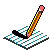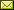PHYSICS@HORTONPhysics12

Physics 11

Math 11

NS Exams

Curriculum

Tips

Tutorials

McGraw Hill

Resources

Need it?

News

Enrichment

GiancoliMATH11

April 2012 Notes

 April 3 CONCEPT CHECK: Sinusoidal Models with Equation GROUP WORK: Sinusoidal Model (with equation) PRACTICE: Solving Trigonometric Equations April 5 TEST OUTLINE (continued) (answers) Practice Test (Interpreting Graphs & Sinusoidal Functions) (answers) April 11 TEST April 13 Interpreting Graphs Example Stats Intro & Review PRACTICE: Interpreting Graphs April 17 Measures of Spread & Distribution PRACTICE: p176/1-4 (answers) Notes April 19 Statitical Measure & Histograms (using the calculator) GROUPWORK:Measures of Central Tendancy, Histograms & Measure of Spread Notes April 23 Normal Curve & percentages under curve PRACTICE: Normal Curve (answers) on-line practice Notes April 25 z-scores GROUP WORK: Normal Curve Notes